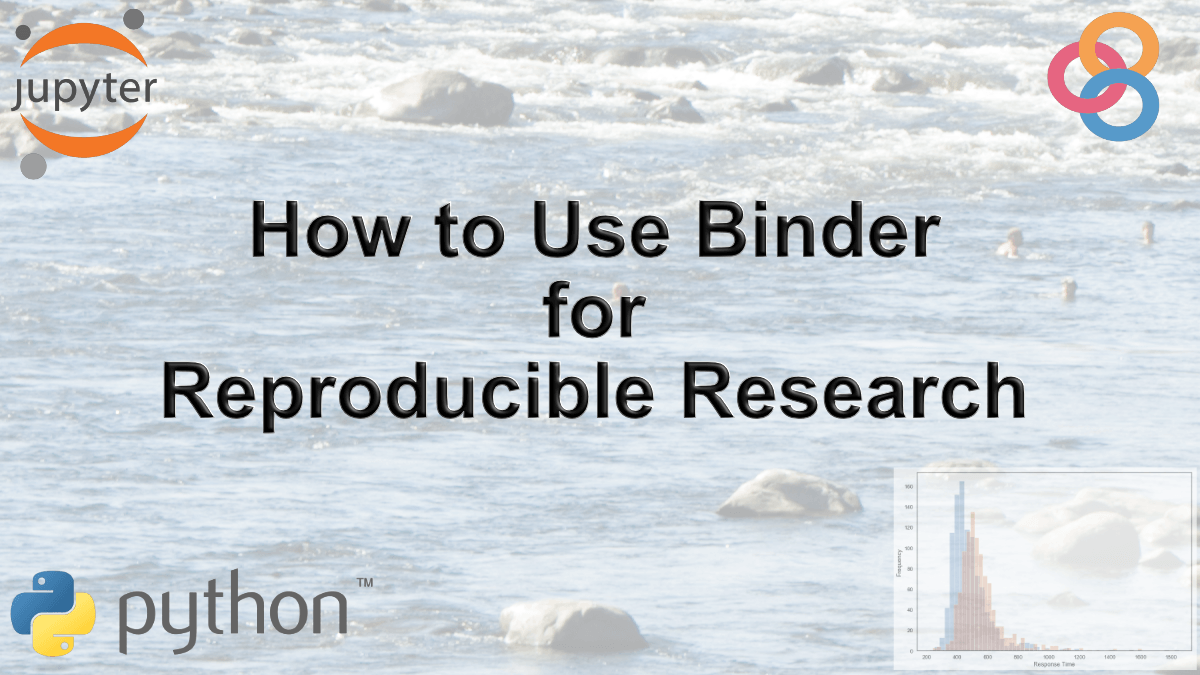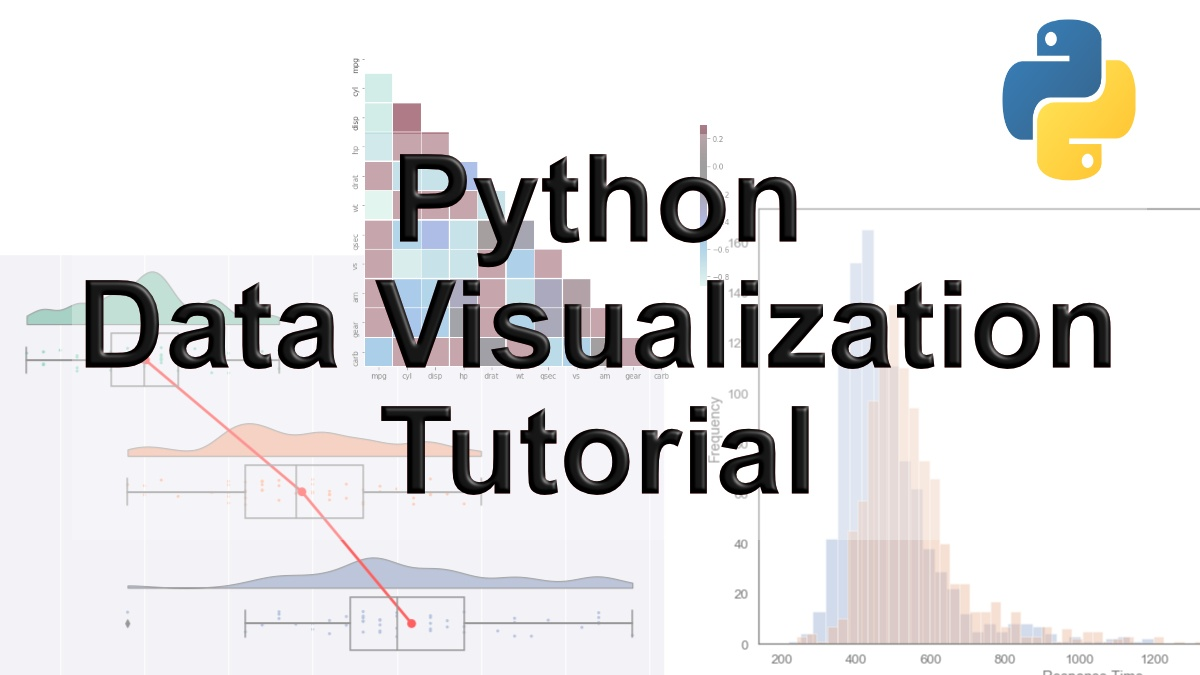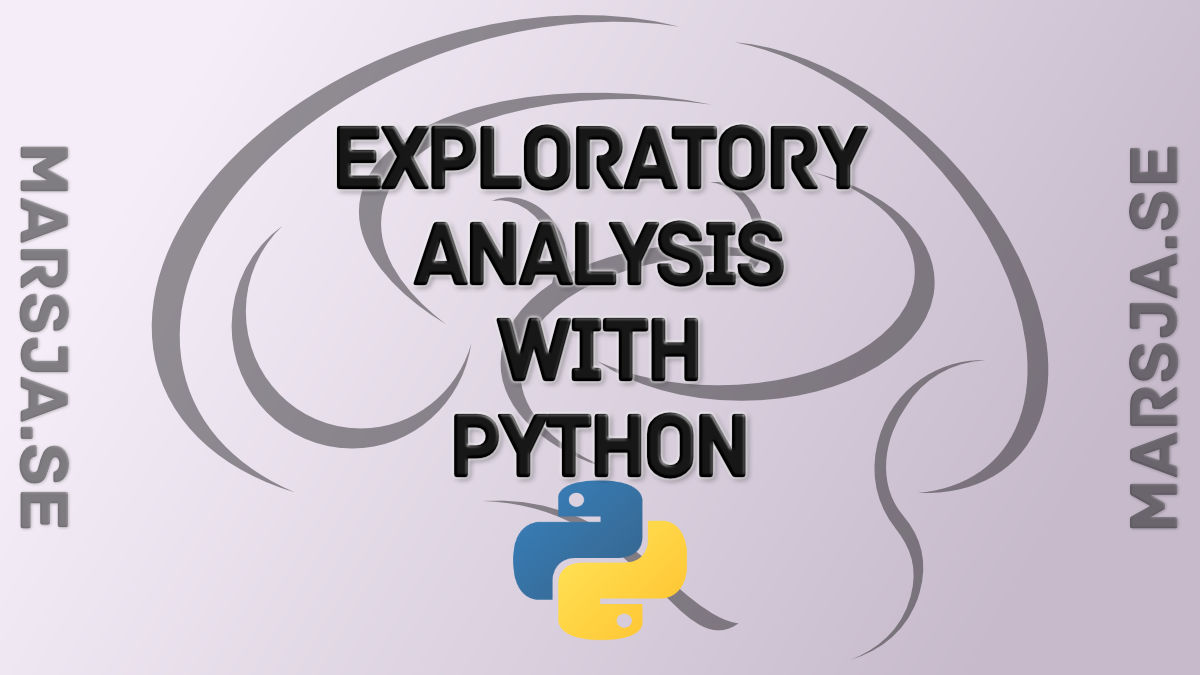## How to Use Binder and Python for Reproducible Research

In this post, we will learn how to create a binder so that our data analysis, for instance, can be fully reproduced by other researchers. That is, here we will learn how to use a tool called Binder for reproducible research. In previous posts, we have learned how to...## 9 Data Visualization Techniques You Should Learn in Python

With the ever-increasing volume of data, it is impossible to tell stories without visualizations. Data visualization is an art of how to turn numbers into useful knowledge. Using Python we can learn how to create data visualizations and present data in Python using...## OpenSesame Tutorial: How to use Image Stimuli

In this OpenSesame tutoria,l we will learn how to use images as stimuli and how to load the trials; including filenames, correct responses, and conditions from a pre-generated CSV file. To follow this tutorial you don’t need to know Python programming. However,...## Exploratory Data Analysis in Python Using Pandas, SciPy, and Seaborn

In this post, we are going to learn how to explore data using Python, Pandas, and Seaborn. The data we are going to explore is data from a Wikipedia article. In this post, we are actually going to learn how to parse data from a URL using Python Pandas. Furthermore, we...